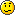grams to liters

Forum rules
Dear convert-me.com forum visitors,

Our forum has been available for many years. In September 2014 we decided to switch it to read-only mode. Month after month we saw less posts with questions and answers from real people and more spam posts. We were spending more and more resources cleaning the spam until there were less them 1 legitimate message per 100 spam posts. Then we decided it's time to stop.

All the posts in the forum will be available and searchable. We understand there are a lot of useful information and we aren't going to remove anything. As for the new questions, you can always ask them on convert-me.com FaceBook page

Thank you for being with us and sorry for any inconveniences this could caused.

grams to liters

I need to convert 50g into liters. Is the following correct: 50g = 50cm^3 = 50 ml = .050L?
kp

The weight to volume ratio above is correct ONLY if using a substance that has a specific gravity of 1.0, such as water at 4Âş C.

However, notwithstanding specific gravity, 50 cm^3 = 50 ml = 0.05 L
Dirtman
Expert

Posts: 574
Joined: Sat Jan 21, 2006 2:04 pm
Location: California

Many thanks. I tried to look up specific gravity in my textbook (I'm in Freshman Inorganic chemistry) and it's not there so I guess I'm not supposed to know that yet.

What I'm trying to do is find the work done when 50 grams of tin dissolves in excess acid at 1 atm and 25 C. I know that work=-(P)(deltaV). In similar problems in the chapter, when we didn't know volume, we calculated it using V=nRT/P. However, in all those problems, the products & reactants were liquids and/or gasses with mass given in liters. For this problem, the initial volume is given in grams. I'm assuming I should use the same approach, i.e. calculate V-final and V-initial, determine delta V, and solve.

Would anyone be willing to comment on whether or not this is the right approach?
kp

conversions

you have no idea how long ive been looking for that conversion thanks
crackerjack

Since you have a pressure and volume, it's likely that they want you to convert your grams to moles (n) and use VP=nRT. Then take you liters (V) and apply to your equation. Because 50g does not equal 50 cm^3. Those are entirely separate. Mass and Volume. There is no conversion. (Unless you've got density)(density=g/L) Although it does beg the question how my teacher expects me to find grams of O2 from 2.54 liters without any other variables.obblman

• List of all units you can convert online
• Metric conversion
• Convert pounds to gallons
• Convert grams to cups
• Grams to milliliters
• Imperial vs US Customary
• History of measurement